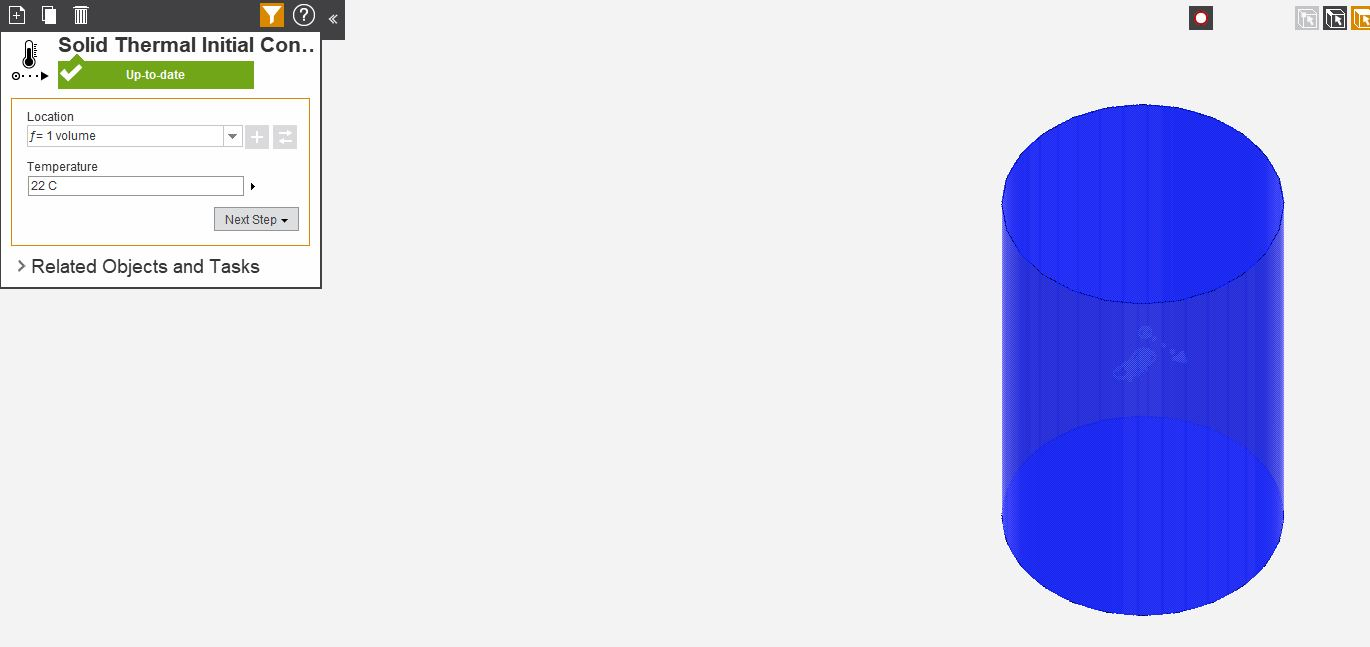## Fluids

•AnthonyL
Subscriber

Hello,

I would like to set the initial condition of my model as a function in order to simulate the cooling of a cylinder with heterogeneous temperature.

The temperature of each point of the solid should be given by something like this:

f( sqrt((x)^2+(y)^2+(z)^2)) )

Where f is a user defined function and x,y,z are the coordinates of the point in 3D, in my case it is a text file containing temperature as a function of distance.

In Ansys AIM I can't find an option to set the temperature other than a fixed homogeneous temperature (see attached picture)

Any idea on how to proceed ?

Thanks

Anthony•rachels1001
Subscriber

Hey AnthonyL,

I'd suggest creating a UDF to input this as a boundary condition.

Once you have that UDF, you can create a for loop that loops over the cells in the face. You can then define a coordinate system to the face, and define the temperature accordingly. So for example:

face_t f;

real temper = 0.0;

real xL,yL,zL     /*this defines coordinate system variables for you */

real xC{ND_ND];    /*this defines the array that the coordinate system function will fill for you */

{

xL = xc;

yL = xc;

zL = xc; /*this retrieves the array values as variables you can now work with */

temper = sqrt((xL)^2 + (yL)^2 + (zL)^2)); /*this defines a temperature value based on the centroid coordinates */

after this point you would need a function that could define the temperature of the cell in that face, I don't know one off the top of my head but there should be one within the documentations, you would just use the temper variable you initialized earlier.

Does anyone else know a function that does this?

This is just a basic guess on my part, but let me know if this helps!

•rachels1001
Subscriber

So as an update, you could use the F_Profile function to define the temperature of the individual cell. Below is a sample UDF that does the same thing with pressure:

``` DEFINE_PROFILE(pressure_profile,t,i)
{
real x[ND_ND];    /* this will hold the position vector */
real y;
face_t f;
begin_f_loop(f,t)
{
F_CENTROID(x,f,t);
y = x;
F_PROFILE(f,t,i) = 1.1e5 - y*y/(.0745*.0745)*0.1e5;
}
end_f_loop(f,t)
}```
•AnthonyL
Subscriber

I managed to set up the temperature as a function of position for my model with the UDF you provided above,

I also switched to fluent to solve the transient thermal problem

One thing that confuses me is if I set that function as boundary condition won't it be applied at all times ? I only want this function a t=0s the rest of the time the temperature is mostly controlled by radiation and thermal conduction.

•AnthonyL
Subscriber

Ok So I used your code above and changed DEFINE_PROFILE to DEFINE_INIT which is run once at the beginning of the simulation.

This gives me the case needed.